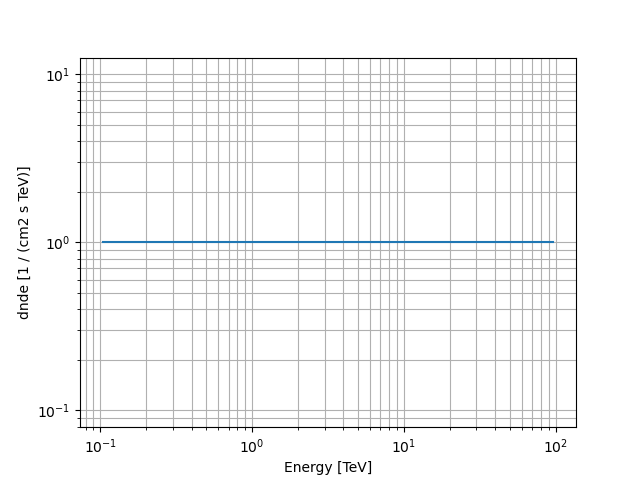# Constant spectral model¶

This model takes a constant value along the spectral range.

$\phi(E) = k$

## Example plot¶

Here is an example plot of the model:

from astropy import units as u
import matplotlib.pyplot as plt
from gammapy.modeling.models import ConstantSpectralModel, Models, SkyModel

energy_bounds = [0.1, 100] * u.TeV
model = ConstantSpectralModel(const="1 / (cm2 s TeV)")
model.plot(energy_bounds)
plt.grid(which="both")## YAML representation¶

Here is an example YAML file using the model:

model = SkyModel(spectral_model=model, name="constant-model")
models = Models([model])

print(models.to_yaml())


Out:

components:
-   name: constant-model
type: SkyModel
spectral:
type: ConstantSpectralModel
parameters:
-   name: const
value: 1.0
unit: cm-2 s-1 TeV-1


Gallery generated by Sphinx-Gallery